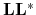Madagascar Programming Reference ManualNext: The dot-product test Up: Introduction Previous: Products of operators

A very important notion in data processing is the adjoint operatorof an operator. In the discrete world, the adjoint operator ofis its (conjugate) transpose, i.e.. From this definition of the adjoint it is evident that the adjoint of the adjoint is the original operator (since). Consider a vectorand the adjoint of the zero-padding operator,. ThenWe conclude that the adjoint of data zero-padding is data truncation. It is also easy (but tedious) to verify that the adjoint operation of the convolution betweenandis the crosscorrelation ofwith. One may also notice that for the specific zero-padding operator,, i.e. in this case the adjoint neutralizes the effect of the operator. It is tempting to say that the adjoint operation is the inverse operation, however this is not the case: it is not always the case that. In fact such an equality is meaningless mathematically ifis not a square matrix (ifis amatrix, thenis, whileis).is not even the left inverse of: notice that in the case of the zero padding operator,(the lastelements ofare zero). However it is often the case that the adjoint is an adequate. It is the case though quite often that the adjoint is adequate approximation to the inverse (sometimes within a scaling factor) and it is also quite probable that the adjoint will do a better job than the inverse in inverse problems. This is because the adjoint operator tolerates data imperfections, which the inverse does not.

From the definition of the adjoint operation as the left multiplication the complex conjugate matrix, it follows that the adjoint of the product of two linear operators equals the product of the adjoints in reverse order, i.e.. This is naturally extended to the product of any finite product of operators, i.e.. The adjoint of the product is also implemented in [sec:chain]chain.c.Madagascar Programming Reference ManualNext: The dot-product test Up: Introduction Previous: Products of operators

2011-07-02